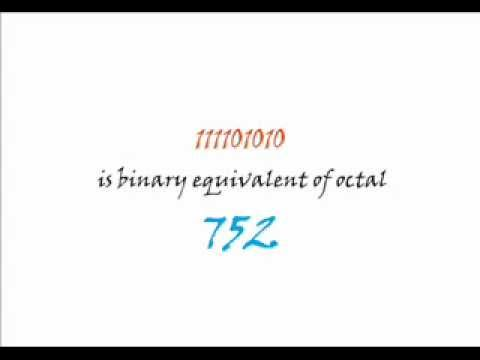## Octal Number System

Subject: Computer Science

#### Overview

The number with base eight is called octal number. We can generate these numbers with the combination of 0, 1, 2 3, 4, 5, 6, 7. This note provides an information about Octal Number System and its conversions.
##### Octal Number System

The number with base eight is called octal number. It is represented by Q or O. We can generate these numbers with the combination of 0, 1, 2 3, 4, 5, 6, 7. We can represent these numbers wit suffix eight. Eg (5432)8Weighted value

 85 84 83 82 81 80 32768 4096 512 64 8 1

#### Conversions from Decimal to Octal

Decimal number is repetitively divided by eight and remainders are arranged in the form of octal numbers.

Example

Convert (240)10into octal.

 8 240 0 8 30 6 3

(240)10= (360)8

Convert (356)10into octal.8 356 4 8 44 4 4

(365)10=(444)8

#### Conversions from Octal to Decimal.

Each octal is multiplied by its weighted position. The sum of all products is known as decimal form of octal.

Example

Convert the octal numbers into decimal

1. (340)8= 3 x 82+ 4 x 81+ 0 x 80=192 + 32 + 0 = (224)10
2. (175)8= 1 x82+7 x 81+ 5 x80= (125)10

#### Octal to Binary and Binary to Octal Conversions

The three digit format of binary digits is used for octal to binary conversions or vice versa.

#### Octal to Binary

3-bits binary numbers are written for each octal digit.

Example

Convert (56)8 (octal) into binary.

Algorithm:

• Convert each octal digit into binary and make three digits grouping.
5= 101
6= 110

Now our equivalent binary number is (56)8=(101110)2

Convert (64102)8 into Binary number.

6= 110
4= 100
1= 001
0= 000
2= 010

Now our equivalent binary number is (64102)8= (110100001000010)2

#### Binary to Octal

The binary numbers are broken into 3-bits section from last bit and convert into octal equivalent of each binary section. Table shows the decimal, octal and equivalent binary bits.

 Decimal Octal Binary 0 0 000 1 1 001 2 2 010 3 3 011 4 4 100 5 5 101 6 6 110 7 7 111

Example

Convert (10011)2 into octal number.

Algorithm

• make 3-bits grouping from last bit.
010 011
• convert each group into decimal numbers.
010 = 2
011 = 3
= (23)8

Convert (1011010011)2 into octal number.

001 011 010 011

001= 1
011= 3
010= 2
011= 3

= (1323)8

Algorithm

• Convert octal into binary.
• make groups of 4-bits from last bit.
• convert each group into decimal numbers.

Example

• Convert into binary
3= 011, 4= 100, 7=111, 6= 110, 5=101
(011100111110101)2
• Make a four digits group from the last bit of the binary number.
0011 1001 1111 0101 (Add 0 before the group to make four digit)
3 9 15 5 (Convert into equivalent decimal numbers)
=(39F5)16Where F= 15
##### Things to remember
• The number with base eight is called octal number. It is represented by Q or O.
• When decimal number is repetitively divided by eight and remainders are arranged in the form of octal numbers,then decimal number are converted into octal.
• Each octal is multiplied by its weighted position. The sum of all products is known as decimal form of octal.
• The three digit format of binary digits is used for octal to binary conversions or vice versa.
• It includes every relationship which established among the people.
• There can be more than one community in a society. Community smaller than society.
• It is a network of social relationships which cannot see or touched.
• common interests and common objectives are not necessary for society.
##### Videos for Octal Number System##### Binary to octal and octal to binary conversion##### Octal to Decimal Shortcut/Easy Conversion

A number system that uses eight different digits to represent different values is known as octal number system. The base of octal number system is 8 because it consist eight digits from 0 to 7. The octal number system is used in the computer to represent binary numbers. The place values in the octal number can be expressed by using powers of 8.

The procedure for conversion of octal numbers to their decimal equivalents is similar to conversion of binary numbers to their decimal equivalents but with the difference, that the base used in this case is 8 instead of 2 used in binary.

Solution:

3 2 1 0 (weight)

Octal Number: 5 2 6 4

Decimal Equivalent: 5×83 + 2×82 + 6×81 + 4×80

= 5×512 + 2×64 + 6×8 + 4×1

= 2560 + 128 + 48 + 4

= 2740

Hence, (5264)8 = (2740)10

Octal digit is represented in 3 bits. An octal number is converted to its binary equivalent by just substituting the respective binary value for each digit of the octal number.

Binary Table

 Octal Binary 0 000 1 001 2 010 3 011 4 100 5 101 6 110 7 111

Solution:

Octal Number: 3 2 0 6

Binary Equivalent: 11 010 000 110 (From Binary Table)

Hence, (3206)8 = (11010000110)2

There is no any direct method to convert octal number into octal. So, at first the given octal number is converted into its binary equivalent then the result will be converted into hexadecimal as in previous methods.

Octal Number: 3 2 5 6

Binary Equivalent: 11 010 101 110 (From Binary Table)

Again,